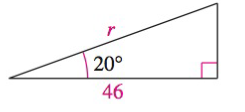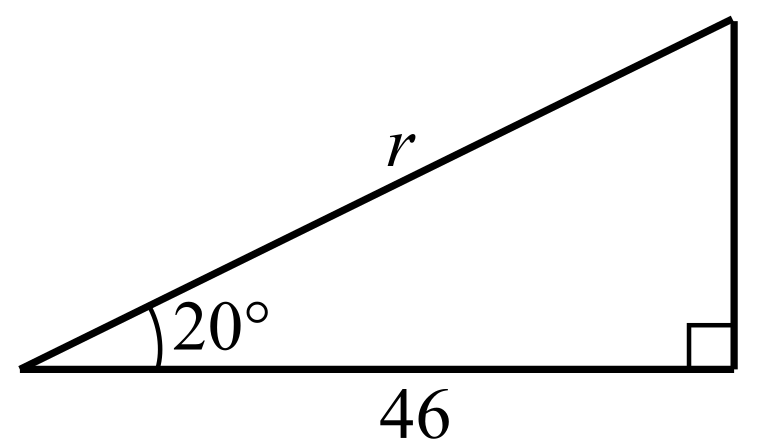Chapter 8.2, Problem 48E### Calculus: An Applied Approach (Min...

10th Edition
Ron Larson
ISBN: 9781305860919

#### Solutions

Chapter
Section### Calculus: An Applied Approach (Min...

10th Edition
Ron Larson
ISBN: 9781305860919
Textbook Problem
1 views

# Solving a Right Triangle In Exercises 43-48, solve for x, y, or r as indicated. SeeSolve for r.To determine

To calculate: The value of r in the provided right angle triangle.Explanation

Given Information:

The provided triangle is shown below:

Formula used:

cos20°0.9396

Right triangle definition:

sinθ=yr         cscθ=rycosθ=xr         secθ=rxtanθ=yx         cotθ=xy

Where, x is the length of the side adjacent to θ, y is the length of the side opposite to θ, and r is the length of hypotenuse

### Still sussing out bartleby?

Check out a sample textbook solution.

See a sample solution

#### The Solution to Your Study Problems

Bartleby provides explanations to thousands of textbook problems written by our experts, many with advanced degrees!

Get Started

#### Identify the quadratic polynomial from among (a)-(c) and evaluate it using.

Mathematical Applications for the Management, Life, and Social Sciences

#### Calculate y'. 24. y=1/x+x3

Single Variable Calculus: Early Transcendentals

#### Find for x = 3t2 + 1, y = t6 + 6t5. t4 + 5t3 4t3 + 15t2

Study Guide for Stewart's Single Variable Calculus: Early Transcendentals, 8th